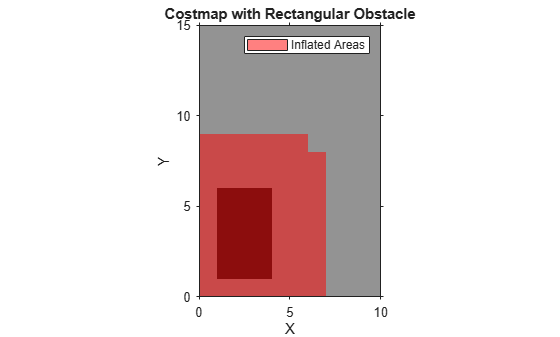# setCosts

Set cost value of cells in vehicle costmap

## Syntax

``setCosts(costmap,xyPoints,costVals)``

## Description

example

````setCosts(costmap,xyPoints,costVals)` sets the costs, `costVals`, for the (x, y) points in `xyPoints` in the vehicle costmap.```

## Examples

collapse all

Create a 10-by-15 meter vehicle costmap. Cells have a side length of 1 meter.

`costmap = vehicleCostmap(10,15);`

Define a set of (x,y) coordinates that correspond to a 3-by-5 meter rectangle.

```[x,y] = meshgrid(2:4,2:6); xyPoints = [x(:),y(:)];```

Mark the rectangle as an obstacle by increasing the cost of its cells to 0.9.

```costVal = 0.9; setCosts(costmap,xyPoints,costVal); plot(costmap) title('Costmap with Rectangular Obstacle')```## Input Arguments

collapse all

Costmap, specified as a `vehicleCostmap` object.

Points, specified as an M-by-2 real-valued matrix that represents the (x, y) coordinates of M points.

Example: `[3.4 2.6]` specifies a single point at (3.4, 2.6)

Example: `[3 2;3 3;4 7]` specifies three points: (3, 2), (3, 3), and (4, 7)

Cost of points in `xyPoints`, specified as an M-element real-valued vector.

Example: `0.8` specifies the cost of a single point

Example: `[0.2 0.5 0.8]` specifies the cost of three points

## Version History

Introduced in R2018a